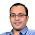### Another Coin Problem

Jaadu asked me this question in a Convex Optimization Class this semester. I was able to solve it. He said it was in one of Diwan Sir tutorials. :)
Interesting puzzle!!

You are given N coins (assume N to be of the form 2^k). Some are light and other are heavy.You are given one weighing machine. What is the number of weighing required to find the number of coins that are heavy?

A hint:
Try to divide the coins in two equal parts such that each have both have same number of heavy coins.

Coins Puzzle
Five Thieves and Bounty

Update (11/12/11): Weighing machine here is a weighing balance. Its a function isXgtY {Input X: Set of Weights, Input Y: Set of Weights, Output Bool 1 if X>Y and 0 if X<=Y}

1.Divide a set of 2^n coins with k heavy coins into two sets, each with 2^(n-1) coins with floor(k/2) heavy coins. If we can do this, we can determine the number of heavy coins in O(log n) operations.

Dividing the set can be done in O(log n). (*Later)

So, T(n) = T(n/2) + O(log n)
T(n) = O(log^2 n).

(*Sub-Algorithm)
Divide the set into two sets A and B of equal number of coins. Let A greater than B and to make both side of equal weight, I need to shift few coins from A to B and equal number of coins from B to A. So divide A into A1 and A2, and B into B1 and B2. Move A2 from A into B and B1 from B into A now if (A1,B1) is greater than (B2,A2) then I have not moved enough coins from A into B so as to make B part heavy enough hence you divide A1 into A11,A12 and move A12 in B side similarly u move B21 into A side on the other hand if (A1,B1) is less than (A2,B2) I have more than enough coin from A into B hence move B12 back into B and A21 back into A now measure again. Do it so on...

Note that we are doing this in O(log n) as each time the number of coins we are moving is reduced by half. So, In O(log n), we are done.

To prove that solution always exists, we do it by induction:
difference in the number of heavy coin after kth iteration cannot be more than 2^(n-1-k)
So, after n-1th iteration, it can be more that 1 coin. Hence, done. :)

1.How do you determine the number of heavy coins at the end? What is the stop criteria when there is an odd number of heavy coins?

2.How do you determine the number of heavy coins in the end? What is the stop criteria for your dividing algo if there is an odd number of heavy coins?

2.An alternate solution:

start by weighing 1 coin - it might be the lighter or the heavier. And then weigh all the coins together - this will tell us whether the first weight is for the lighter coin or the heavier coin.

If Coin-1 is lighter, divide the pile into two halves and weigh one of them to chose the heavier half. Keep splitting the halves obtained into two and proceeding with the heavier half until you have only one coin which will be the heavier coin. Knowing both the weights, the numbers can be easily found.

Chose the lighter halves if the first coin obtained was heavier.

This will take a maximum of (n+2) weighings.

3.